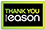# Computational Electronic Circuits

Hardback
This textbook teaches in one, coherent presentation the three distinct topics of analysis of electronic circuits, mathematical numerical algorithms and coding in a software such as MATLAB®. By combining the capabilities of circuit simulators and mathematical software, the author teaches key concepts of circuit analysis and algorithms, using a modern approach. The DC, Transient, AC, Noise and behavioral analyses are implemented in MATLAB to study the complete characteristics of a variety of electronic circuits, such as amplifiers, rectifiers, hysteresis circuits, harmonic traps and passes, polyphaser filters, directional couplers, electro-static discharge and piezoelectric crystals. This book teaches basic and advanced circuit analysis, by incorporating algorithms and simulations that teach readers how to develop their own simulators and fully characterize and design electronic circuits. Teaches students and practitioners DC, AC, Transient, Noise and Behavioral analyses using MATLAB;Shows readers how to create their own complete simulator in MATLAB by adding materials learned in all 6 chapters of the book;Balances theory, math and analysis;Introduces many examples such as noise minimization, parameter optimization, power splitters, harmonic traps and passes, directional couplers, polyphase filters and electro-static discharge that are hardly referenced in other textbooks;Teaches how to create the fundamental analysis functions such as linear and nonlinear equation solvers, determinant calculation, random number generation and Fast Fourier transformation rather than using the built-in native MATLAB codes.
€90.99272 Reward Points
In stock online
Delivery in 2-3 working days

Any purchases for more than €10 are eligible for free delivery anywhere in the UK or Ireland!

This textbook teaches in one, coherent presentation the three distinct topics of analysis of electronic circuits, mathematical numerical algorithms and coding in a software such as MATLAB®. By combining the capabilities of circuit simulators and mathematical software, the author teaches key concepts of circuit analysis and algorithms, using a modern approach. The DC, Transient, AC, Noise and behavioral analyses are implemented in MATLAB to study the complete characteristics of a variety of electronic circuits, such as amplifiers, rectifiers, hysteresis circuits, harmonic traps and passes, polyphaser filters, directional couplers, electro-static discharge and piezoelectric crystals. This book teaches basic and advanced circuit analysis, by incorporating algorithms and simulations that teach readers how to develop their own simulators and fully characterize and design electronic circuits. Teaches students and practitioners DC, AC, Transient, Noise and Behavioral analyses using MATLAB;Shows readers how to create their own complete simulator in MATLAB by adding materials learned in all 6 chapters of the book;Balances theory, math and analysis;Introduces many examples such as noise minimization, parameter optimization, power splitters, harmonic traps and passes, directional couplers, polyphase filters and electro-static discharge that are hardly referenced in other textbooks;Teaches how to create the fundamental analysis functions such as linear and nonlinear equation solvers, determinant calculation, random number generation and Fast Fourier transformation rather than using the built-in native MATLAB codes.
Quantity:
In stock online
Delivery in 2-3 working days272 Reward Points

Any purchases for more than €10 are eligible for free delivery anywhere in the UK or Ireland!

€90.99
In stock online
Delivery in 2-3 working days